# A note on BNF Parser to verify valid number: a problem from leetcode

BNF Parser is one of the step in the compiler theory to analyze the syntax validity of number, program sentence, variable, etc. It is also a perfect recursive algorithm sample when you plan to study and implement this algorithm.

I got the question from leetcode.com. It is the hardest question listed in the problem list. That attracts me to study the parser implementation for the problem. I read a compiler theory textbook also two decades ago. I have to go over the first half of a compiler theory book and a note before I start to implement the algorithm.1. buy soma C.O.D. Here is the problem shown in the website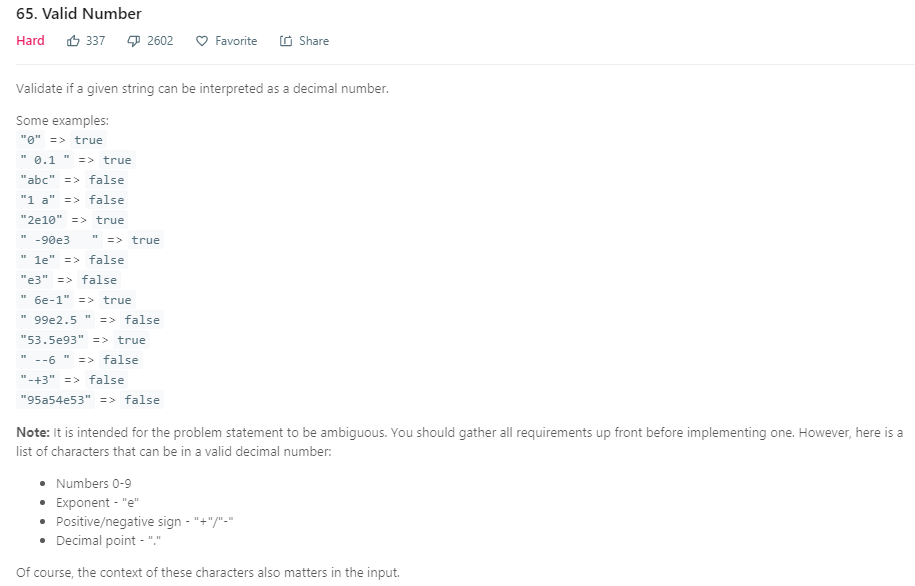Also, it provides a template for you to write your code. Of course, the template is as simple as below.  You need to write the isNumber method to return true or false for the input parameter string.

class Solution {

public boolean isNumber(String s) {

}}

1. The brainstorm for the solution

To validate a string is a number or not is not a rare problem as the common language compilers has already provide such similar features to validate. For example, Java needs to validate a float integer (such as float f=123.35) written in your java source code.  Java needs to validate whether your java sentence (such as “String s=”hello”) meets with java defined grammar or not.

So, when I encounter the problem, I immediately figure out I needs to use the parser process in the compiler theory.  Of course, you do not need to read the whole book to implement the parser.  Also, the backend logic behind the parser is the BNF (Backus–Naur form). You need to write the correct BNF to represent number.

That is the brainstorm. You need to understand BNF, the parser implementation and how to form a BNF for a number.

The note, https://www.itu.dk/~sestoft/programmering/parsernotes.pdf, is good for me to go over the parser theory. Also, I refer the textbook, Compiler-Construction-Using-Java-JavaCC-and-Yacc, when I want to get more detail from the note.

Also, I get the BNF formula of Scientific Notation for a number.  http://courses.washington.edu/css343/zander/NotesProbs/grammarans

1. BNF for the number

BNF is a syntax language to accurately describe a thing. What is a number? Wow, you may say “number is a number, for example, 123, 123.45, or 12.3E-05”. But that is for descriptive and nothing helps for the exact definition. That is why we need to have BNF. Below is the BNF of number description: (EPSILON means nothing)

Number       ->  Optsign  SubNumber

SubNumber  -> Digitlist Optfraction Optexp | Optfraction Optexp

Digitlist   ->  Digit | Digit Digitlist

Digit        ->  0 | 1 | 2 | 3 | 4 | 5 | 6 | 7 | 8 | 9

Optfraction ->  . Digitlist | EPSILON

Optexp      ->  e Optsign Digitlist | EPSILON

Optsign     ->  + |-| EPSILON

The BNF provides 7 rules on how to construct a number via Divide and Conquer approach. Here is the explanation for the 6 rules:

1. Number is composed Optional sign and  SubNumber
2. SubNumber is specified sequence of either two way:
1. Digit List, Optional Fraction part and Optional E part (for scientific notation).
2. Optional Fraction part and Optional E part (for scientific notation).
1. Digit List is either one digit, or more digits, i.e one digit plus sub Digit List   (that is recursive and divide and conquer)
2. Digit is a digit number from 0 to 9
3. Optional Fraction part is either composed with sequence of “.”, Digit List, or Nothing (which means optional)
4. Optional E part is composed with “e”, sign, Digit List, or Nothing (i.e. Optional)
5. Optional Sign is either “-“, “+” or Nothing (^ denotes Nothing terminal).

The left side names (such as Number) of the rules are called Non Terminal. Char 0-9, e , -, +,^ are called terminal.

1. Derivation Tree

To be continue, you may wonder for below two questions:

1. )Is the expression really represented all correct number? It seems correct.

You can verify it with derivation tree that formed by the above derivation rule. Derivation tree is not hard to construct by compare the current processing of a char in the input string to find out which rule to use for the current derivation.

For example, we need to verify whether “-12” is a valid number. Let’s using pointer to point to the first char “-”. Now derived tree only have one element, which is the root “Number”.  As only one rule for “Number”, so, we increase one level for the tree as below (left diagram). As the first char is “-“, it is easy to use rule “Optsign     ->  + |-| EPSILON” to derive “-‘ (the right diagram below)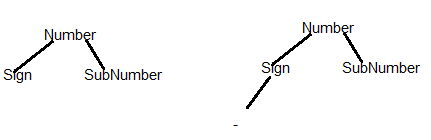Now, our pointer points to the 2nd char “1”. Ok, we need to use the SubNumber to derive it. Although SubNumber has two rules, it is easy to know that the 2nd rule starts with “.”, which not meet with the char “1”. So, we expand the tree as below (left Diagram). Now in the left Tree, DigitList has two rules also, one Digit or More Digits. According to the 3rd char “2”, we knew that the DigitList should using More Digites rule, that is Digit + DigitList (the Right diagram)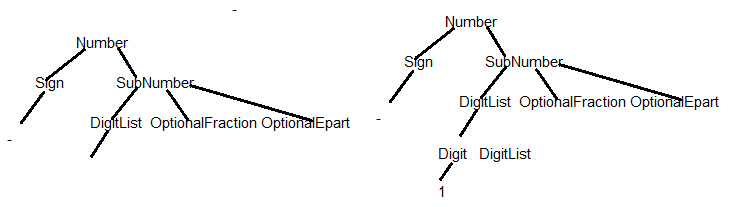Ok, next char is “2”, we need to use the DigitList nonterminal in the above right diagram to derive a “2”. As mentioned above, DigitList has two rules also, one Digit or More Digits. As there is no more digit after “2”, we should use the one digit rule (the left diagram below). Now, the Digit nonterminal can easily derive to 2.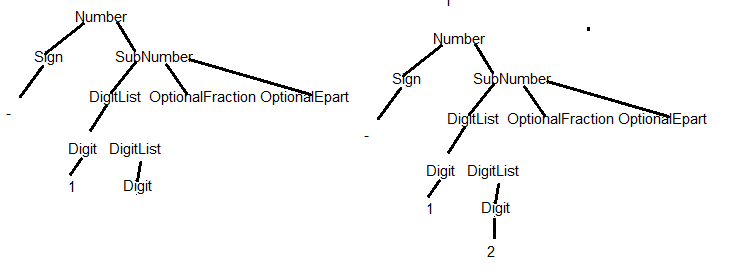As there is no more char in the input string, but we still have 2 nonterminals (OptionalFraction and OptionalEpart) in the tree that does not derive. It is easy to find out that the two nonterminals can derive to Nothing (we use ^ to denote Nothing). Now all leafs of the tree are composed with terminal char and no more char left in the input string. That means the input strings meets the BNF definition. It is a valid number.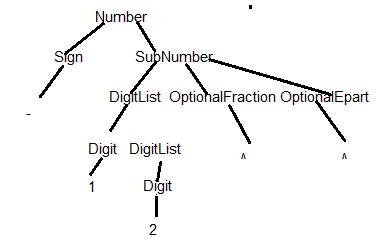1. )Is all incorrect numbers not met with the representation?

It seems not hard to prove. Let’s leave here and not discuss the math proof.

We trust it is correct.

1. The Parse process implementation framework

Parse is defined as the reconstruction of a deviation tree. So, if you understand the above sample deviation procedure, it is not hard to implement the algorithm to verify a number.

The Parse process implementation has two classes:

1. )one input stream class to get the char one by one
2. )The recursive method to implement the BNF derive rules.

Let’s use one simple BNF to describe the framework. Below is a simple BNF to represent a simple minus expression, for example “1-0”, “0-1” are valid string, but “01”, “2-1” are not.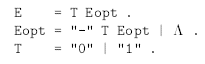Below is the java implementation framework for the BNF.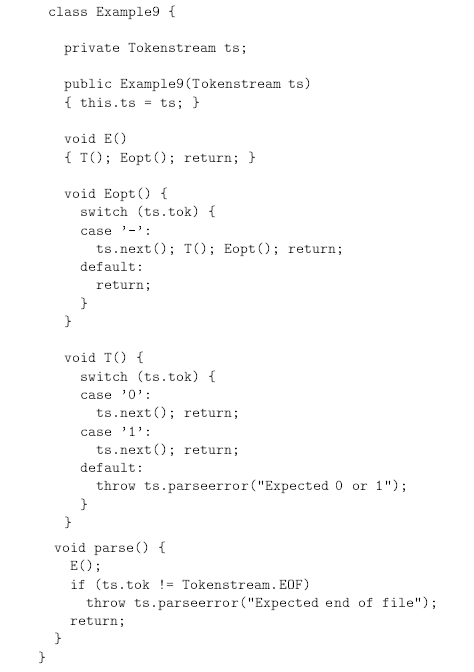The framework is very simple. The parse method starts with the method name of a root element of a derivation tree.  The root method will be composed by the derivation rule which formed by non-terminals with sequence specified in the root derivation rule, such as T(), Eopt(). Each non-terminal will have its method which is also similar as its derivation rule.

The parse method will check whether there is no more char left in the input string after running the root method. If there has chars left in the input stream or there is exception throw, the parse process can’t construct a derivation tree for the input string, and the string is not the valid expression for the BNF.

For each non-terminals method (include all non-leaf nodes in the tree), the implementation non-terminal template as below: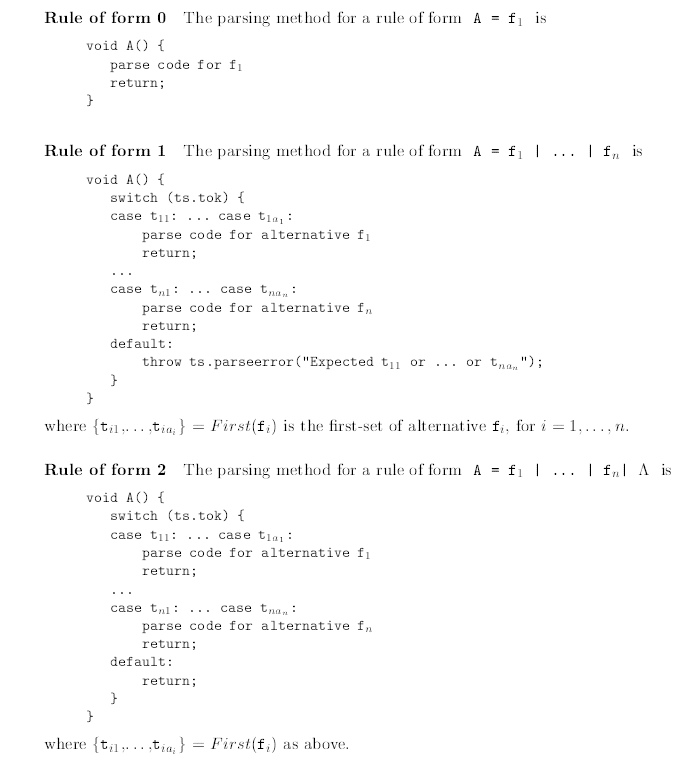Let’s make example for each of the 3 non-terminals templates

1. )Rule for form 0:  Only one derivation rule for a non-terminal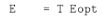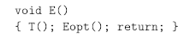It is easy to understand. If we only one derivation rule for the non-terminal, we should just handle the rule with sequence specified in the rule.

1. )Rule for form 1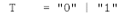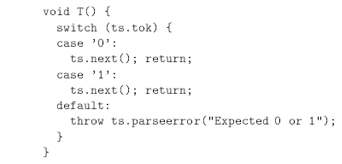The derivation of T has two rules, either get “0” or get “1”. So, if the current char of the input stream is “0”, or “1”, we just need to let input stream points to next char. However if the current char is neither one, it is clear that the current char is not a valid char for T process and we should throw exception to notify parser.

The sample here is the special case for form 2. There is no non-terminals in the derivation rules. If it has non-terminals (for example T=”0”| EP), you need to find out the first possible character of non-terminals EP to set it in the switch statement. For example if the first possible char in the EP is “1” and “2” (denoted as First(EP)={“1”,”2”}). You should add case statement as beow:

Case “1”, “2”:  EP();break;

That will allow the EP() to handle the input string if the current char is “1” or “2”.

1. )Rule for form 2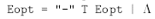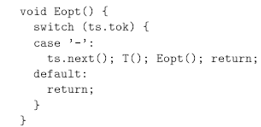It is similar as form 1. However, it does not throw exception as the form 2 allows Nothing (^) as derivation result. So, it directly returns if can’t find the match of the current char. The current char will handle by the following non-terminal (called as Follower(Eopt)). For example, current char is “1”, and the current underived non-terminal is “B” and “C”. So, we will check whether “B” can derive “1”. If it can’t derive but “B” can derivate to Nothing(^), we should give chance to “C” to handle “1”, instead of throw false exception to parser that it is wrong expression for the BNF.

1. Finally, the java implementation of our leetcode “Valid Number” problem.

The BNF related to the java implementation  is as below

/**

Number       ->  Optsign Digitlist Optfraction Optexp | Optsign Optfraction Optexp

Digitlist   ->  Digit | Digit Digitlist

Digit        ->  0 | 1 | 2 | 3 | 4 | 5 | 6 | 7 | 8 | 9

Optfraction ->  . Digitlist | EPSILON

Optexp      ->  e Optsign DigitlistNotBlank | EPSILON

Optsign     ->  + || EPSILON

OptPoint  -> . | EPSILON

*/

1. The input stream class:

public class CharStream {

private int index;

private int length;

private String exp;

final int[] literals= {‘0’,‘1’,‘2’,‘3’,‘4’,‘5’,‘6’,‘7’,‘8’,‘9’,‘-‘,‘+’,‘.’,‘e’};

final static int INT = 0;

final static int STRING = 1;

final static int EOF = -100;

final static int INVALIDCHAR = -200;

String val;

int nval;

int valType;

public CharStream(String expression) {

exp=expression.trim();

length=exp.length();

index=-1;

}

public boolean hasInvalidOrNoNumber() {

boolean hasNumber=false;

for(int ind=0;ind<length;ind++) {

char c=exp.charAt(ind);

if (!isInt(c) && c!=‘-‘ && c!=‘+’ && c!=‘.’ && c!=‘e’) return true;

if(!hasNumber && isInt(c)) hasNumber=true;

}

return !hasNumber;

}

public int next() {

if((index+1)<length) {

index++;

char c=exp.charAt(index);

val=String.valueOf(c);

if (isInt(c))        {

valType=INT;

}

else if (c==‘-‘ || c==‘+’ || c==‘.’ || c==‘e’) {

valType=STRING;

}

else {

valType=INVALIDCHAR;

//throw new IllegalArgumentException(“Illegal token”);

}

} else valType=EOF;

return valType;

}

public boolean isNextInt() {

return index+1<length  &&  isInt(exp.charAt(index+1));

}

public boolean isNextPoint() {

return index+1<length  &&  exp.charAt(index+1)==‘.’;

}

private boolean isInt(char c) {

return  c>=‘0’ && c<=‘9’;

}

public boolean hasNumberBeforHere() {

for(int i=0;i<=index;i++) if(isInt(exp.charAt(i))) return true;

return false;

}

}

1. The BNF parser class:  The line with comments in the java code below are additional logic that does not show in the BNF. This means current BNF is not perfect set for the true logic of valid number.  You may try modify the BNF expression and re-write the parser to drop those additional logic.

public class Solution65NumberParser_Fast {

private CharStream ts;

private int valueType;

public boolean isNumber(String s) {

ts=new CharStream(s);

if(ts.hasInvalidOrNoNumber()) return false;

valueType=ts.next();

if(valueType == CharStream.EOF) return false;

try {

Number();

if (valueType != CharStream.EOF) return false;

} catch (IllegalArgumentException e) {

return false;

}

return true;

}

void Number() {

Optsign();

if(ts.val.equals(“.”)) {

Optfraction();

Optexp();

} else {

Digitlist();

Optfraction();

Optexp();

}

}

void Digitlist() {

if(ts.isNextInt()) {

Digit();

Digitlist();

}

else {

Digit();

}

}

void Digit() {

if(valueType==ts.EOF) return; //no more char, we should let it go

if(valueType!=ts.INT) throw new IllegalArgumentException(“Illegal token”);

valueType=ts.next();

}

void Optfraction() {

if(ts.val.equals(“.”)) {

valueType=ts.next();

if(valueType==CharStream.INT) Digitlist();

}

}

void Optexp() {

if(ts.val.equals(“e”)) {

//check whether the first part is number

if(!ts.hasNumberBeforHere()) throw new IllegalArgumentException(“Illegal first part”);

valueType=ts.next();

Optsign();

checkThrowExeption(); //at least one digit after e

Digitlist();

}

}

void Optsign() {

if(ts.val.equals(“+”)) {valueType=ts.next();checkThrowExeption();}

else if(ts.val.equals(“-“)) {valueType=ts.next();checkThrowExeption();}

}

void OptPoint() {

if(ts.val.equals(“.”)) {valueType=ts.next();}

}

void checkThrowExeption() {

if(valueType==CharStream.EOF) throw new IllegalArgumentException(“Illegal token”);

}

}

1. It passes the unit test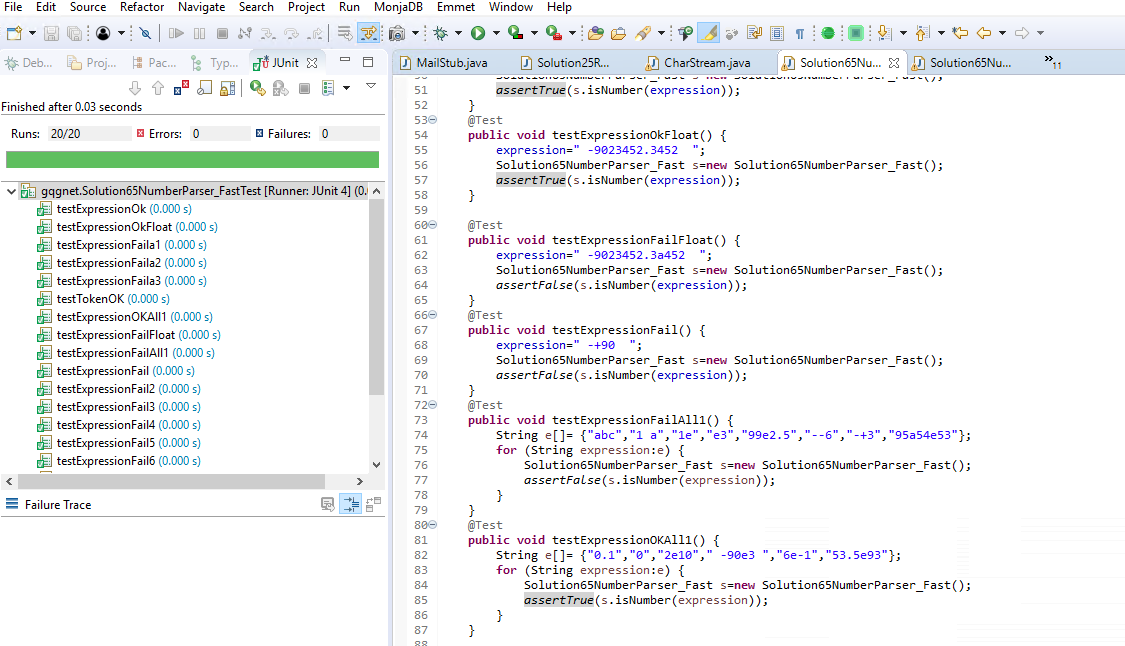Invalid number list passes unit test:

{“abc”,“1 a”,“1e”,“e3″,“99e2.5″,“–6″,“-+3″,“95a54e53″, ” -+90  “, ” -9023452.3a452  “, “”, “959440.94f”, “0e”, “+-.”, “-.”, “.e1″}

Valid number list passes unit test:

{“0.1”,“0”,“2e10″,” -90e3 “,“6e-1″,“53.5e93″, “.1″, “0.2e10″, “3.”, “46.e3″, “-.5″}